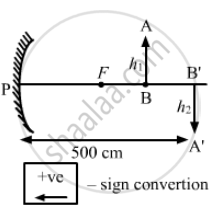Department of Pre-University Education, KarnatakaPUC Karnataka Science Class 12
Advertisement Remove all ads

# A Concave Mirror Forms an Image of 20 Cm High Object on a Screen Placed 5.0 M Away from the Mirror. the Height of the Image is 50 Cm. Find the Focal Length - Physics

Sum

A concave mirror forms an image of 20 cm high object on a screen placed 5.0 m away from the mirror. The height of the image is 50 cm. Find the focal length of the mirror and the distance between the mirror and the object.

Advertisement Remove all ads

#### Solution

Given,
Height of the object, h1 = 20 cm,
Distance of image from screen v = −5.0 m = −500 cm,$- \frac{v}{u} = \frac{h_2}{h_1}$

$or \frac{- ( - 500)}{u} = \frac{50}{20}$
Where 'u' is the distance of object from screen.
(As the image is inverted)
Using mirror formula,

$\frac{1}{v} + \frac{1}{u} = \frac{1}{f}$

$or \frac{1}{- 5} + \frac{1}{- 2} = \frac{1}{f}$

$or - \frac{1}{f} = \frac{7}{10}$

$or f = - \frac{10}{7} = - 1 . 44 \text{ m }$
Hence, the required focal length of the concave mirror is 1.44 m.

Is there an error in this question or solution?
Advertisement Remove all ads

#### APPEARS IN

HC Verma Class 11, 12 Concepts of Physics 1
Chapter 18 Geometrical Optics
Q 2 | Page 412
Advertisement Remove all ads
Advertisement Remove all ads
Share
Notifications

View all notifications

Forgot password?